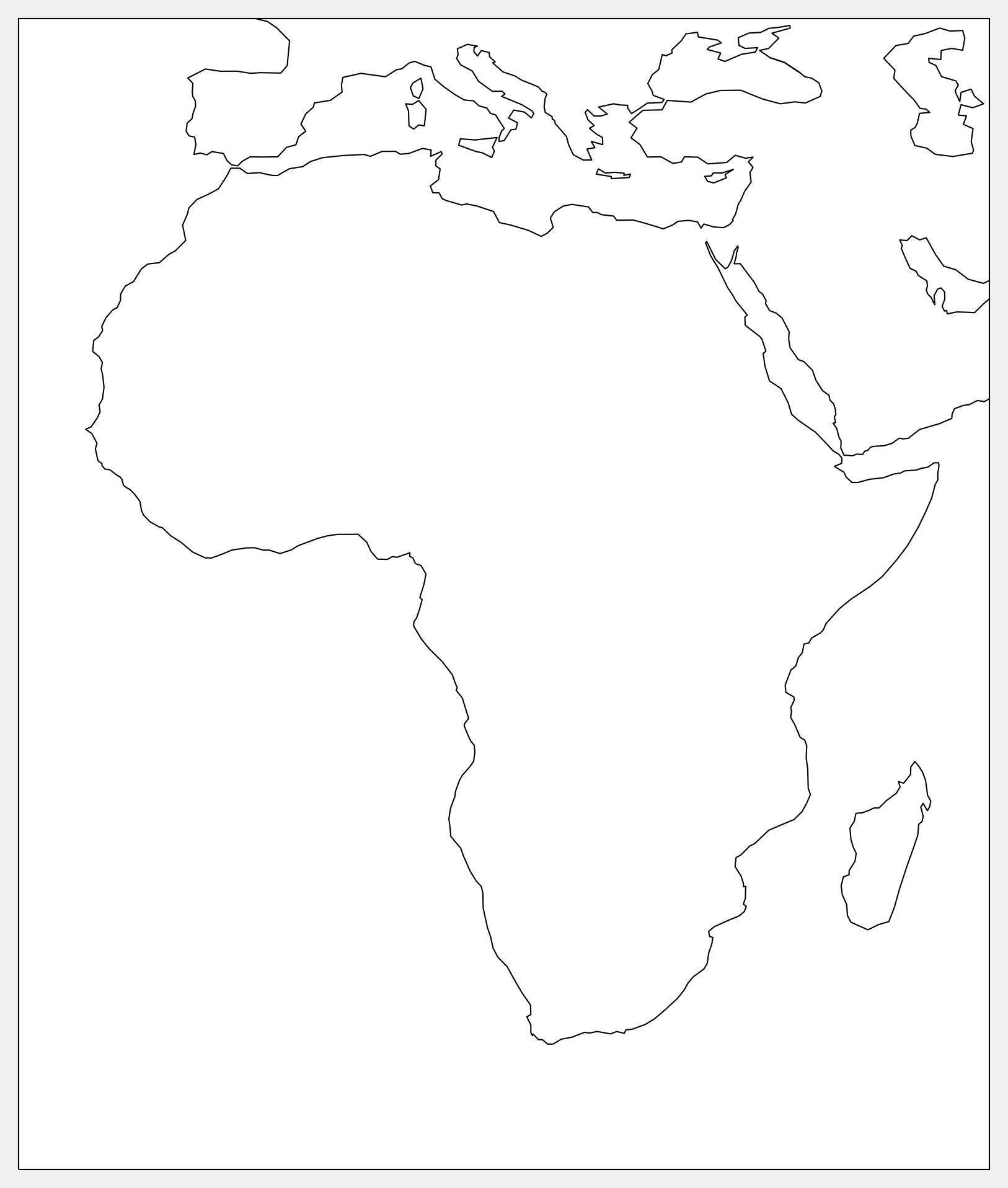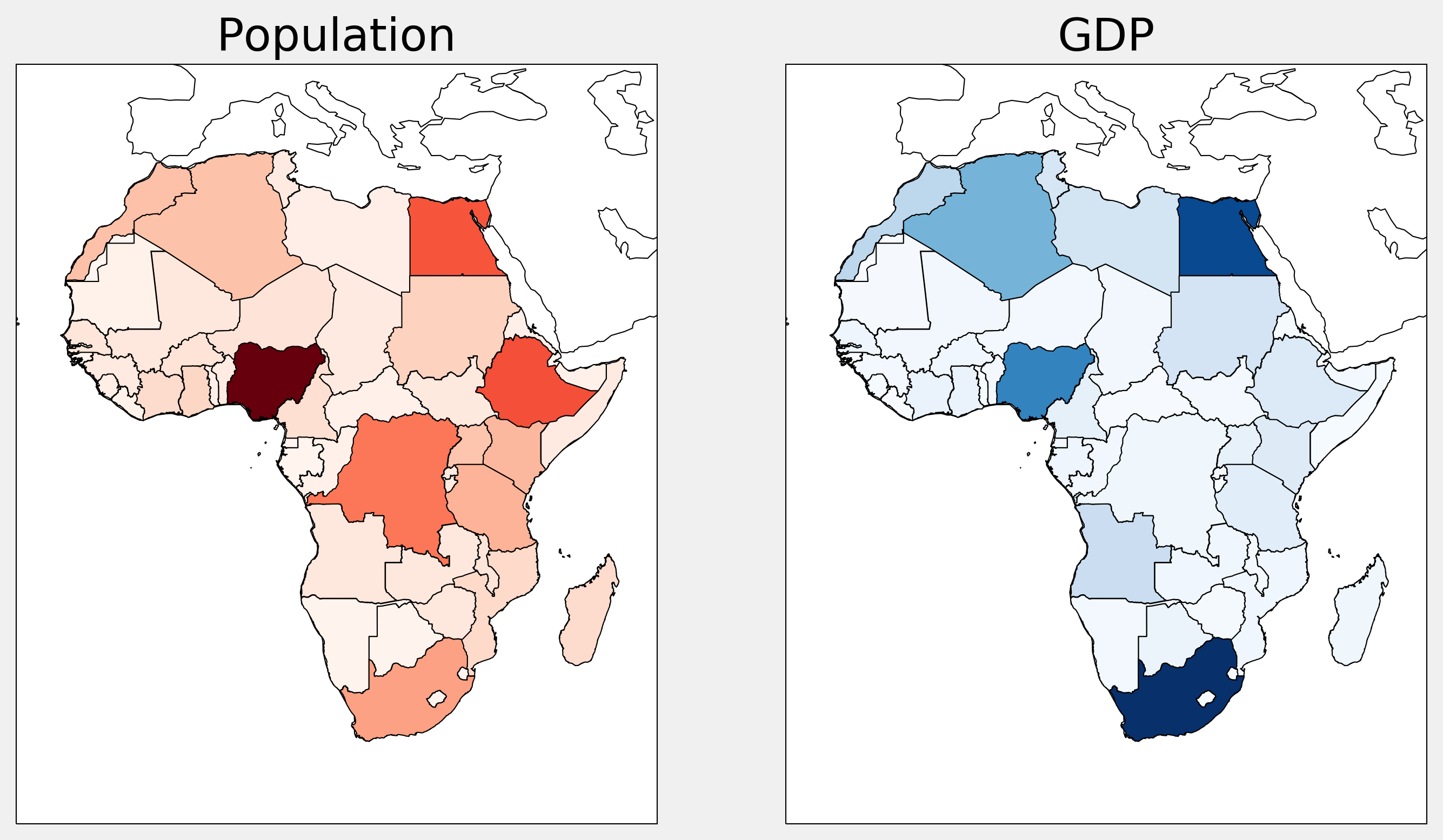This is one of the 100+ free recipes of the IPython Cookbook, Second Edition, by Cyrille Rossant, a guide to numerical computing and data science in the Jupyter Notebook. The ebook and printed book are available for purchase at Packt Publishing.

▶  Text on GitHub with a CC-BY-NC-ND license
▶  Code on GitHub with a MIT license

In this recipe, we will show how to load and display geographical data in the Shapefile format. Specifically, we will use data from Natural Earth (http://www.naturalearthdata.com) to display the countries of Africa, color coded with their population and Gross Domestic Product (GDP). This type of graph is called a choropleth map.

Shapefile (https://en.wikipedia.org/wiki/Shapefile) is a popular geospatial vector data format for GIS software. It can be read by cartopy, a GIS package in Python.

You need cartopy, available at http://scitools.org.uk/cartopy/. You can install it with `conda install -c conda-forge cartopy`.

## How to do it...

1.  Let's import the packages:

```import io
import requests
import zipfile
import numpy as np
import matplotlib.pyplot as plt
import matplotlib.collections as col
from matplotlib.colors import Normalize
import cartopy.crs as ccrs
from cartopy.feature import ShapelyFeature
%matplotlib inline
```

```url = ('https://github.com/ipython-books/'
'cookbook-2nd-data/blob/master/'
'africa.zip?raw=true')
r = io.BytesIO(requests.get(url).content)
zipfile.ZipFile(r).extractall('data')
```

3.  We keep the African countries:

```africa = [c for c in countries.records()
if c.attributes['CONTINENT'] == 'Africa']
```

4.  Let's write a function that draws the borders of Africa:

```crs = ccrs.PlateCarree()
extent = [-23.03, 55.20, -37.72, 40.58]
```
```def draw_africa(ax):
ax.set_extent(extent)
ax.coastlines()
```
```fig, ax = plt.subplots(
1, 1, figsize=(6, 8),
subplot_kw=dict(projection=crs))
draw_africa(ax)
```5.  Now, we write a function that displays the countries of Africa with a color that depends on a specific attribute, like the population or GDP:

```def choropleth(ax, attr, cmap_name):
# We need to normalize the values before we can
# use the colormap.
values = [c.attributes[attr] for c in africa]
norm = Normalize(
vmin=min(values), vmax=max(values))
cmap = plt.cm.get_cmap(cmap_name)
for c in africa:
v = c.attributes[attr]
sp = ShapelyFeature(c.geometry, crs,
edgecolor='k',
facecolor=cmap(norm(v)))
```

6.  Finally, we display two choropleth maps with the population and GDP of all African countries:

```fig, (ax1, ax2) = plt.subplots(
1, 2, figsize=(10, 16),
subplot_kw=dict(projection=crs))
draw_africa(ax1)
choropleth(ax1, 'POP_EST', 'Reds')
ax1.set_title('Population')

draw_africa(ax2)
choropleth(ax2, 'GDP_MD_EST', 'Blues')
ax2.set_title('GDP')
```## There's more...

The geoplot package, available at https://github.com/ResidentMario/geoplot, provides high-level tools to draw choropleth maps and other geospatial figures.